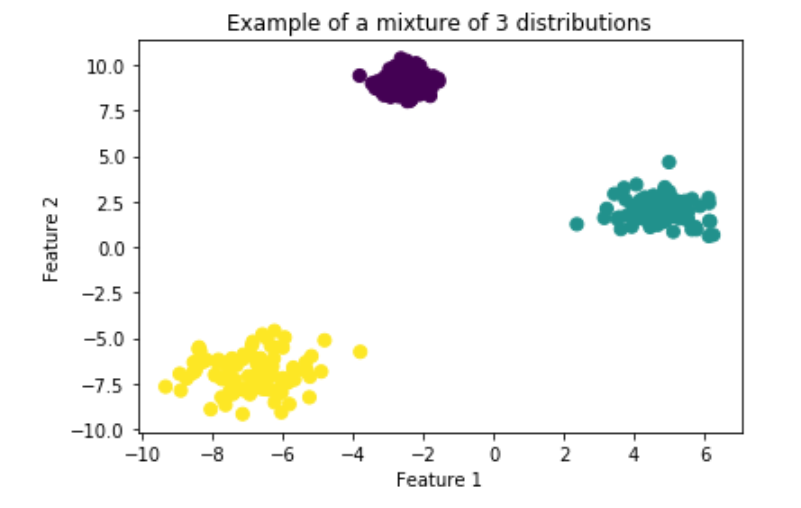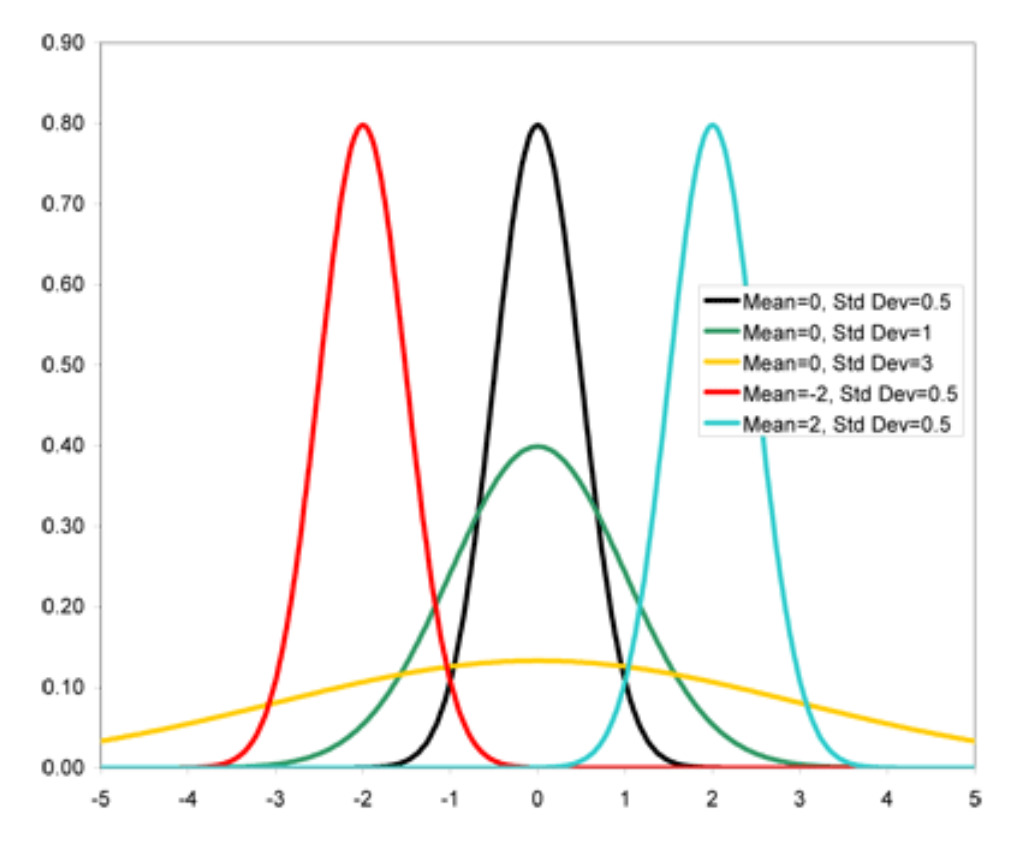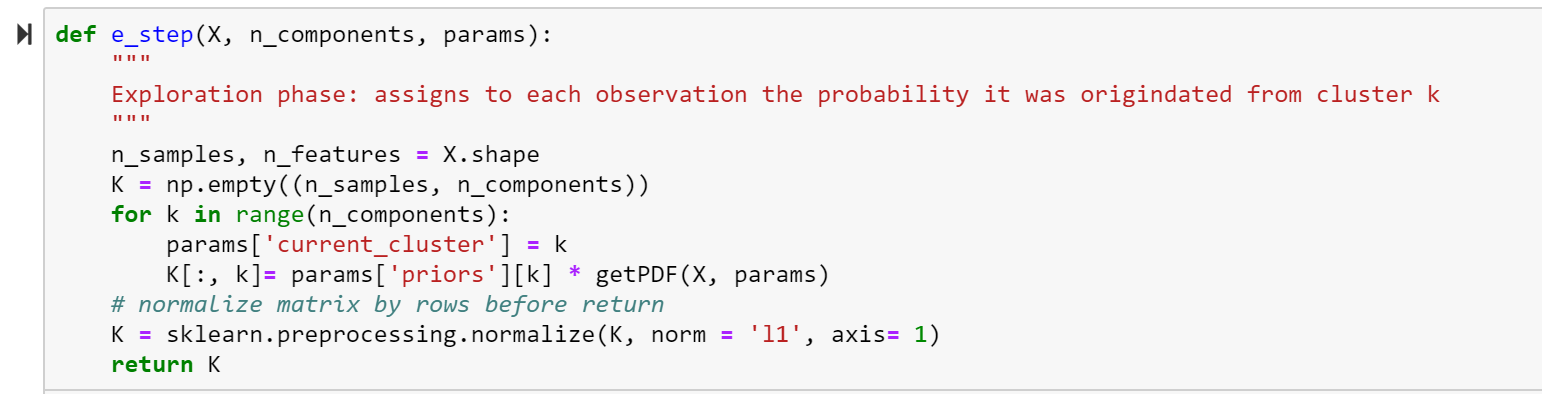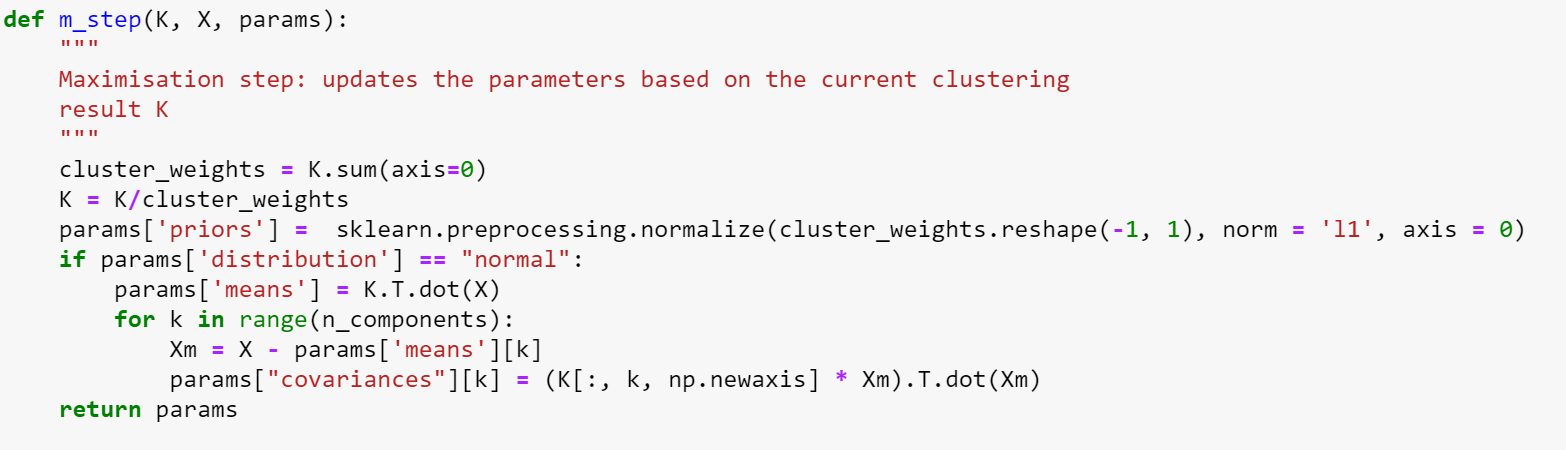Search
• Madalina Ciortan

# Expectation maximization

Finding the Maximum Likelihood Estimate of a model depending on unobserved latent variablesThis article presents a basic python implementation of the expectation maximization algorithm applied to Gaussian distributions. The entire code has been made available as a notebook on Github.

There are datasets consisting of a mixture of distributions (for simplicity, we will consider Gaussians, but the same heuristic can be applied to other types of distributions):

dataset = N(mean1, sd1) + N(mean2, sd2) + … + N(mean_n, sd_n)

where N represents a normal distribution described by a mean and a standard deviation values.

In the example below we generated a dataset containing samples from 3 distributions having random means and the following standard deviations [0.5, 0.7, 1]. We would like to find the parameters of a statistical model which maximize the likelihood that the process described by this model produced the observed data.

```n_components = 3
X, truth = make_blobs(n_samples=300, centers=n_components,
cluster_std = [0.5, 0.7, 1],
random_state=42)```
```plt.scatter(X[:, 0], X[:, 1], s=50, c = truth)
plt.title(f"Example of a mixture of {n_components} distributions")
plt.xlabel("Feature 1")
plt.ylabel("Feature 2");```#### What is the Maximum Likelihood Estimation (MLE)?

MLE is a statistical method for estimating the parameters of a model for a given data. For the sake of clarity, let’s take the most simple example. We observe data coming from only one normal distribution and we would like to determine the parameters (in this case the mean and the standard deviation) which best approximate the data. As we select different values for the mean and for the standard deviation, we get very different results for the probability density function:Thus, we want to calculate and maximize the probability of observing all data, or in statistical term the joint probability distribution of all observations. Then, we make the assumption that each data point is generated independently of the others, which allows us then to calculate the total probability as the product of the marginal probabilities (the probability of observing each point individually). Our desired optimization consists in finding the parameters (mean, sd) which lead to maximum total probability with respect to the observed data.

This comes down to calculating the points where the first derivative is 0. In practice, it is more convenient to work with the natural logarithm of the likelihood function, as this transforms the product into a sum (log-likelihood) and it is easier to differentiate. As the logarithm is a monotonically increasing function, it ensures that the maximum values occur at the same point as in the probability function. In practical terms, it can happen that the derivative of the log-likelihood is too hard or impossible to differentiate by hand, in which case we can still use iterative methods like expectation maximization to find parameter estimates.

Expectation maximization consists in an iterative process looping between 2 steps, called step e (expectation)and step m (maximization). It needs as input the number of components (clusters) expected to be found in the data and also the type of distribution we will use as approximation (in this case normal distributions). In a nutshell, the algorithm can be reduced to the following pseudo-code:

```expectation_maximization(data, n_components):
params = init(data)
while( !convergence)
cluster_probabilities <- e_step(data, n_components, params)
params = m_step(cluster_probabilities, data)```

#### Initialization

In addition to the n_components, this algorithm maintains a list of parameters which are updated at each iteration. These parameters are:

priors: a list of n_components values, representing the probability of a point belonging to the corresponding cluster. Initially we consider that all clusters have equal probability, thus the priors are list of n_components with values 1/n_componentsmeans: the mean value for each of the n_components clusters. Initially we can select a n_components random points as mean valuescovariances: n_components values of covariance matrix corresponding to each cluster. Initially this matrix is assigned to be the Identity matrix.

#### Expectation step

If we consider that the input data X has shape (n_samples, n_features), this step will calculate a cluster probabilities matrix K of shape (n_samples, n_components) providing for each observation the probability (PDF) it originates from each cluster. In other points, it answers the question: for each point, what is the probability that each of the n_components gaussians generated it?The expectation step uses a probability density function specific to the distribution input type:

```def getPDF(X, params):
"""
Probability density function of the input distribution. This is used in the exploration step in order to
calculate for each observation the probability of being observed as part of cluster k.
"""
if params['distribution'] == "normal":
k = params['current_cluster']
return mvn(params["means"][k], params["covariances"][k]).pdf(X)```

#### Maximization step

Given the cluster probabilities matrix calculated in the previous step, we update the mean, prior and covariance parameters to reflect these probabilities.The exit condition (convergence) applies when the difference between the cluster probabilities matrix between iterations becomes negligible (smaller than an arbitrary delta). In order to avoid the algorithm getting stuck if convergence doesn’t occur in a reasonable amount of time, we can also define a maximum number of iterations after which we exit.

```def convergence(K, K_prev, delta):
"""
Check if the algorithm converged by estimating the difference between 2 consecutive solutions. If the matrix norm is smaller than a given delta, we take this as a proof of convergence
"""
if K_prev == None:
return False
return np.linalg.norm(K - K_prev) < delta```

#### Future work

The next planned work consists in enriching this model with other types of distributions.

Another future direction is to build regression functionalities on top of this model which will provide posterior probability estimates for given input.

See All